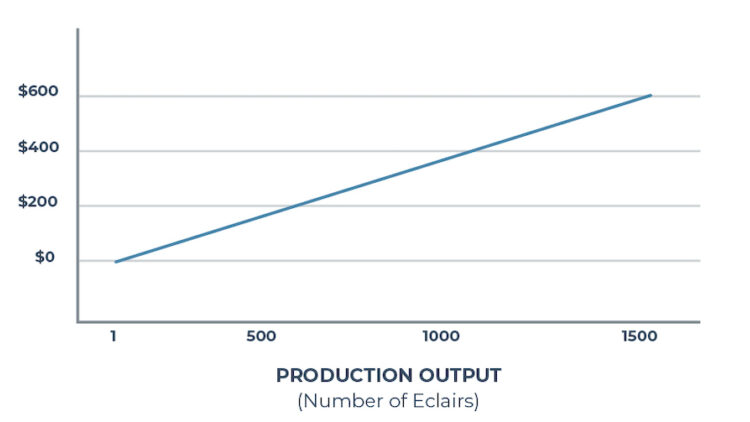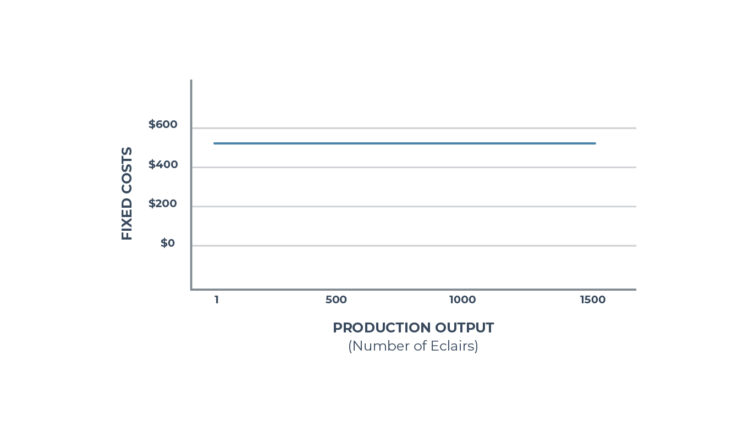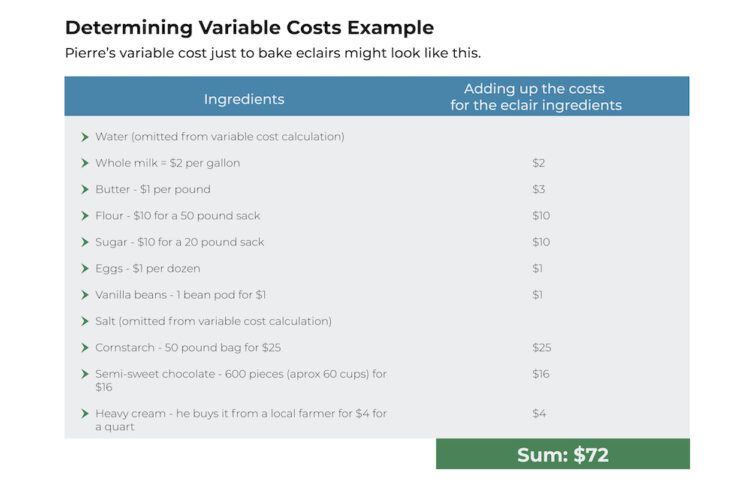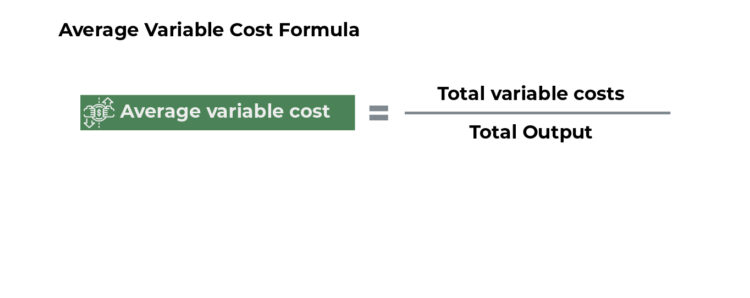## What Is Variable Cost?

Variable costs are the direct costs that a company incurs when producing goods or services.

These costs are directly proportional to the quantity of goods or services produced. As a company’s production output increases, the variable costs increase. As output decreases, variable costs decrease.We’ve included a video that explains variable costs, how to calculate them, and what they include.

### Variable Cost Is Often Confused with...

Variable cost is often confused with variable costing. However, this is an accounting method that’s related to reporting variable costs.

## How to Find Variable Cost

You can calculate variable costs by adding up all of the labor and materials required to produce one unit of anything sold by a company.

### Variable Cost Formula

To find the average variable cost, first calculate the variable cost for all units then divide the sum by the number of units produced:

Total Variable Cost = Total Quantity of Output x Variable Cost Per Unit of Output

But how should you determine the variable cost per unit of output? It depends on what you’re producing and selling.

### Variable Cost Per Unit of Output

Components of variable cost per unit of output may include:

• Direct materials costs

• Direct labor costs

• Transaction fees

• Commissions

• Billable costs

• Utilities

Note: When looking over a list of potential costs to include in the variable cost per unit of output, consider whether the cost changes with the quantity of the output. If so, it’s likely to be a variable cost. If not, it’s probably a fixed cost.

## Example of Variable Cost

As a variable cost example, let’s take Pierre’s French Bakery which is famous for its chocolate cake.

To produce their cakes, Pierre needs the following ingredients:

• Water

• Whole milk

• Butter

• Flour

• Sugar

• Eggs

• Vanilla beans

• Salt

• Cornstarch

• Semi-sweet chocolate

• Heavy cream

Pierre's Bakery also needs utilities such as electricity to run the mixer, water to wash the baking equipment, and natural gas to fire up the ovens. These are the variable costs.

### What Are Fixed Costs?

Pierre’s Bakery must have a mixer, ovens, and other similar items to produce baked goods. Because the cost of equipment remains the same (no matter how many cakes Pierre makes), fixed costs do not change with output.

Other monthly fixed costs might include rent, fire insurance, and equipment rental.

## Variable Costs: Direct vs. Indirect

If costs can be applied directly to the production of a product, they are considered direct costs. For example, Pierre purchases special chocolate to glaze the cakes and the chocolate is only used in his cake production.

At Pierre’s Bakery, indirect costs may include electricity to run the mixers and water used in recipes. Everything Pierre produces uses both electricity and water. The bakery also uses electricity to power the lights and cash register, and water is used in the restrooms. Because the costs of utilities can’t be applied to a specific product, they are considered indirect costs.

## How to Find Variable Costs

Pierre adds up the total cost to make his chocolate cakes. Let’s assume that all of the costs (below) go into each batch.

Pierre’s Bakery uses a great deal of water each month to wash pans and utensils. Therefore, it’s difficult to deduct the exact amount used in recipes from its overall water utility bill. We’ll omit costs such as water because it is an indirect cost and cannot be tied to the production of one single product.

To bake cakes, the variable cost of Pierre’s Bakery might look like this:The variable cost to make all of the cakes is \$72. If Pierre’s recipe makes 6 dozen cakes (72 cakes), the variable cost per unit would be \$1.

Variable cost/total quantity of output = x variable cost per unit of output
Variable cost per unit = = \$72/72 = \$1.

When Pierre puts his cakes in the shop window for sale, he knows he must mark up the cost per cake starting at \$1. However, the \$1 cost per unit only covers his variable costs. He must add the fixed costs per unit (calculated for his entire bakery on an annual basis) to the wholesale cake price. Because the wholesale price was calculated last year, he knows that this figure is \$0.32 cents per item.

By adding the fixed cost (\$0.32) to the variable cost per unit (\$1), Pierre would know the total cost per unit (\$1.32). To make a profit, he must sell each cake for more than \$1.32.

## What Is the Average Variable Cost?

Average variable cost is used to help a company assess whether it will be profitable in the short-run. If marginal revenue (the revenue earned from selling the next unit) remains higher than the average variable cost, the company’s outlook should be positive.

Companies calculate the average variable cost on both a per-item basis and over their entire production line. Once this number is determined, companies can:

• Compare the cost of manufacturing a new product against the company’s average

• Assess the short-run profitability of the company (when taken into consideration with price)

## Average Variable Cost Example

Imagine that a new product has a variable cost of \$4.45 per unit. If the company’s average variable cost for all of its products is \$4.25, the new product’s variable cost should be comparable to the average of the company’s other products.

When the company adds up the variable and fixed costs of producing the new product – and marks up the wholesale production cost by its standard percent – the price of the new product should fit within their product line’s prices (and meet their customers’ pricing expectations).

Imagine that it costs \$7 to produce the new product and the average variable cost for the company’s product line is \$4.25. By the time the company adds up the fixed and variable costs to calculate the wholesale price – then marks up the wholesale price to determine the retail selling price – the new product price may be too high for their customers’ taste.

## How to Use Average Variable Cost

When it comes to applying average variable cost, there’s rarely a one-size-fits-all answer. To determine whether a product should be produced, companies typically run many hypothetical scenarios and examine all factors including competition, market demand, pricing considerations, and their variable and fixed costs.

Due to a higher price, companies may decide that a new product just doesn’t fit into their product line. They may seek new suppliers for the raw materials to make a product for lower costs in order to lower the variable cost and keep production costs in line with their average production costs. If they believe that customer demand will outweigh price considerations, companies may also choose to go ahead and launch the new product at the higher price.

## Average Variable Cost Formula

The average variable cost is calculated by taking a firm’s total variable costs, then dividing it by the total output.

The formula is:To find the total variable cost, look at the variable costing income statement. Otherwise, add the cost of goods sold (COGS) plus the variable selling, general, and administrative expense (SG&A), then divide it by the total output.

At Pierre’s Bakery, the average variable cost includes all of the variable costs incurred by the bakery (e.g. ingredients, packaging, boxes, utilities) divided by the total output of baked goods. To find the average, Pierre must add up the variable costs and divide that sum by the number of baked goods created within a given period, then divide the costs by the quantity.